Introduction to Shape from Shading. Given an image of a uniformly coloured surface, such as the image of a cloth shown below, the human vision system can guess both the shape of the surface and the rough direction of the incident light. This computational inference problem is known as the shape from shading problem.

A standard modelling approach for this problem is to assume the Lambertian reflectance model for the surface and that the surface is lit with a single distant light source. This is a simple computer grahics model, and it allows us to compute a shaded image for any given surface and light source direction. The shape from shading problem is the inverse of this image synthesis process; we wish to find the shape of the 3D surface and the light source direction given the image alone.

In the publication listed further below we explore two new algorithms for shape from shading problems. However, first we show some example results from this paper.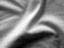Original Cloth Image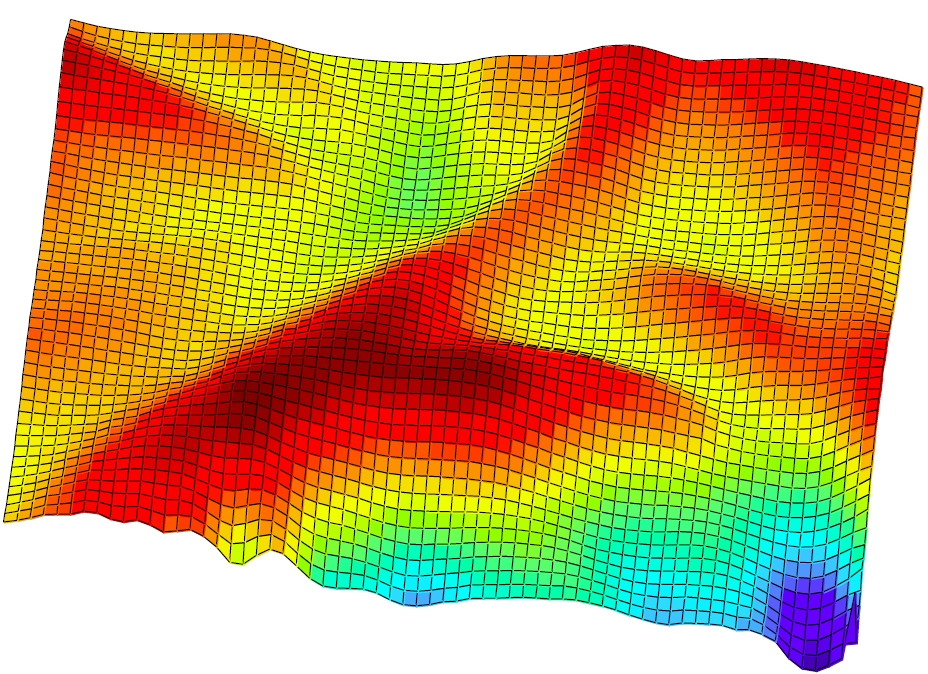Reconstructed Surface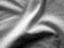Reconstructed Image

Given the image of a loosely folded cloth (top left), a light source direction is computed (not shown) and a surface is reconstructed (right). Assuming a Lambertian reflectance model, this light source and surface can then be used to compute the reconstructed image (bottom left). Note that the recovered surface appears to have a plausible 3D shape and the reconstructed image closely approximates the original image.

A second example is given below. Here the original image is of a hand print in a bowl of granulated sugar. Again the recovered surface is roughly plausible and the reconstructed image closely approximates the original.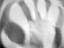Original Sugar Image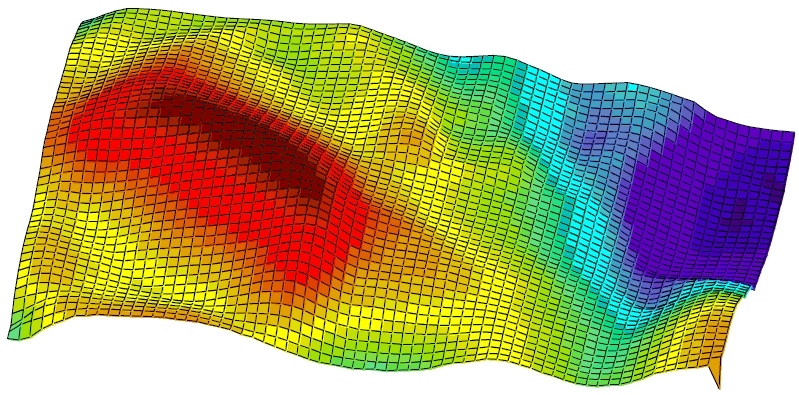Reconstructed Surface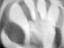Reconstructed ImageAmbiguities in Shape from Shading. In the publication listed below we also show that there is a continuous ambiguity for the recovered surface. That is, even for a fixed light source direction, many different surfaces are rendered to the same image.

One consequence of this ambiguity is that we can demonstrate that your visual system can make a spectacularly incorrect inference of the true 3D surface given only shading information, as shown below.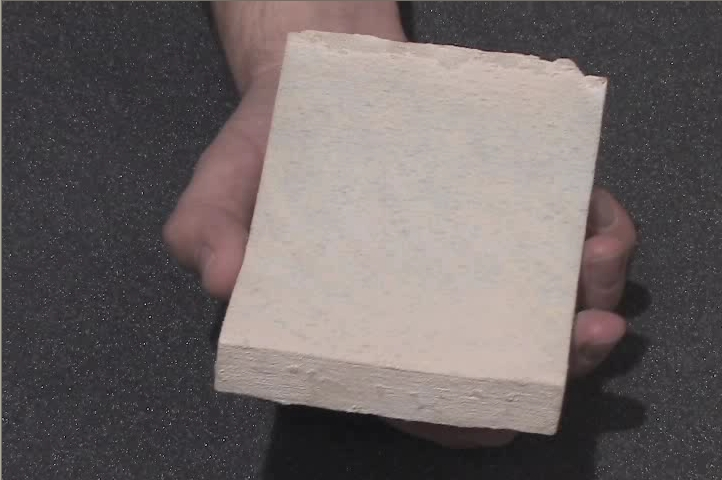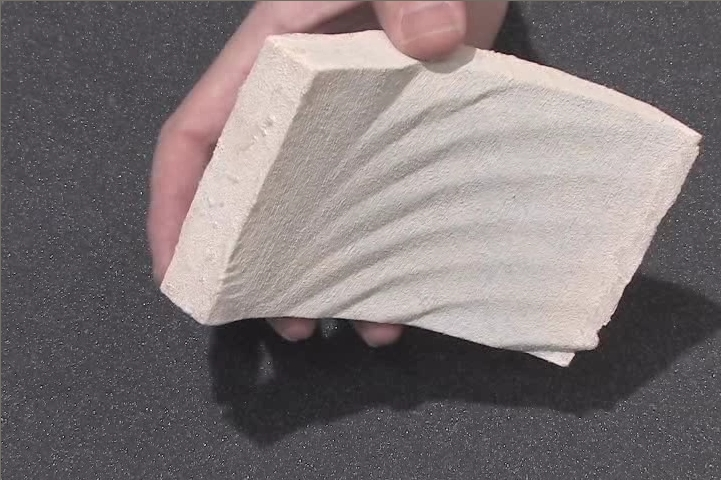Cylinder MPEG-4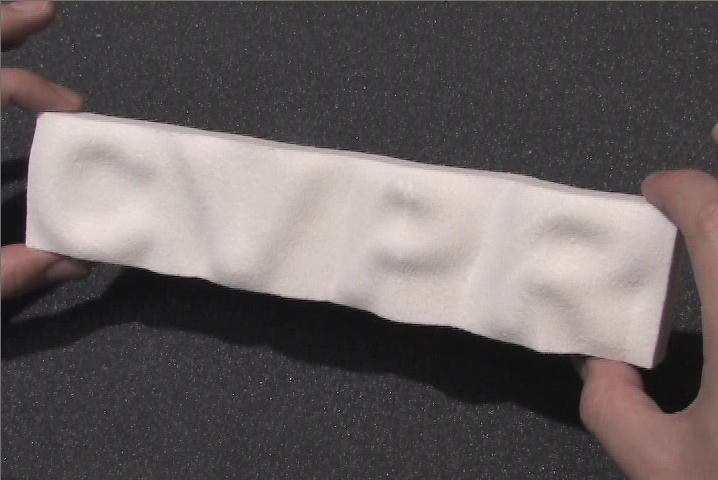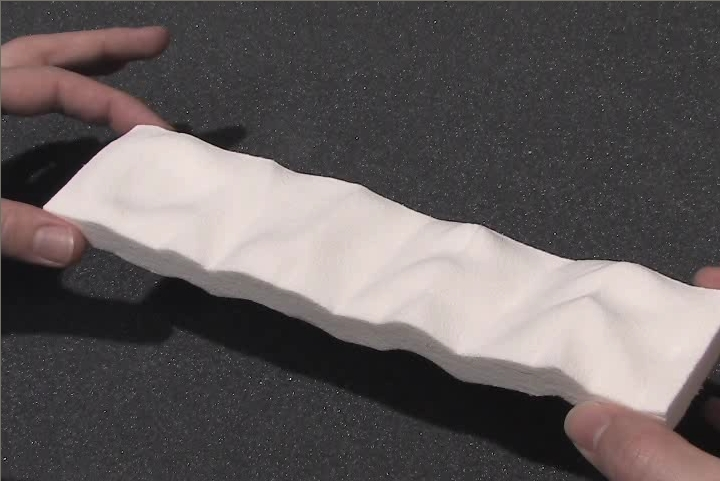CVPR MPEG-4 Shaded Image True 3D Shape

Each row of this table corresponds to a particular rigid 3D object which we pre-computed to exhibit the ambiguity. The desired object was then 3D-printed. When each object is lit from a particular light source direction the shaded image (left) is obtained. Your perception of the underlying surface shape given only this shaded image is very different from the true surface shape (right).

For each row in the table above, use the MPEG-4 link to download a movie which shows the 3D-printed object as it is rotated by hand. The first and last frames of the movie correspond to the two images shown in the corresponding row of the above table.Publication.

Ady Ecker and A.D. Jepson, Polynomial Shape from Shading, Computer Vision and Pattern Recognition (CVPR), San Francisco, USA, June 2010. PDF (5280KB) (Online dataset: Shading test images.)

Summary. This paper discusses two novel computational methods for approximating solutions to the shape from shading problem. One method is based on an optimization strategy using an exact line search, and the second method uses semi-definite programming. The first of these methods was used to compute the 3D surfaces shown above. Moreover, this paper exhibits continuous ambiguities in the recovered surfaces, as illustrated above.